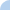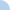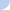Surface Area & Volume of a Cone

If your answer is in linear units you should show units of measure in one of the following ways...
- ft
- feet

If your answer is in square units you should show units of measure in one of the following ways...
- ft²
- square feet
- feet^2
- ft^2

If your answer is in cubic units you should show units of measure in one of the following ways...
- ft³
- cubic feet
- feet^3
- ft^3

If the question asks for an EXACT answer then use pi (and write it as pi); if it does NOT ask for an exact answer, then multiply it out and round your answer to the nearest tenth.Quiz Log In